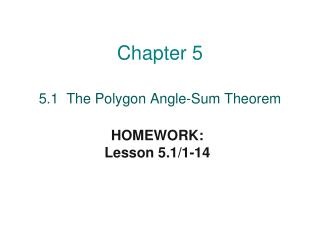DownloadDownload PresentationChapter 5

# Chapter 5

Télécharger la présentation## Chapter 5

- - - - - - - - - - - - - - - - - - - - - - - - - - - E N D - - - - - - - - - - - - - - - - - - - - - - - - - - -
##### Presentation Transcript

1. Chapter 5 HOMEWORK: Lesson 5.1/1-14 5.1 The Polygon Angle-Sum Theorem

2. Objectives • Define polygon, concave / convex polygon, and regular polygon • Find the sum of the measures of interior angles of a polygon

3. Definition of polygon • A polygon is a closed plane figure formed by 3 or more sides that are line segments; • the segments only intersect at endpoints • no adjacent sides are collinear • Polygons are named using letters of consecutive vertices

4. Concave and Convex Polygons • A convex polygon has no diagonal with points outside the polygon • A concave polygon has at least one diagonal with points outside the polygon

5. Regular Polygon Definition • An equilateral polygon has all sides congruent • An equiangular polygon has all angles congruent • A regular polygon is both equilateral and equiangular Note:A regular polygon is always convex

6. Polygon Angle-Sum Theorem The sum of the measures of the angles of an n-gon is SUM = (n-2)180 ex: A pentagon has 5 sides.

7. Sum of Interior Angles in Polygons

8. Sum of Interior Angles Find m∠ X The sum of the measures of the interior angles for a quadrilateral is (4 – 2) * 180 = 360 The marks in the illustration indicate that m∠X = m∠Y = x So the sum of all four interior angles is x + x + 100 + 90 = 360 2 x + 190 = 360 2 x = 170 m∠X = 85

9. Polygon NamesMEMORIZE THESE! 3 sides Triangle 4 sides Quadrilateral 5 sides Pentagon 6 sides Hexagon 7 sides Heptagon 8 sides Octagon 9 sides Nonagon 10 sides Decagon 11 sides Undecagon 12 sides Dodecagon n sides n-gon

10. Naming A Polygon A polygon is named by the number of_____. ex: If a polygon has ___ sides, you use ___ letters. Polygon ABCDE SIDES 5 5

11. Example #1 ABCDEF CDEFAB 1. Name________. Is it concave or convex?__________ 2. Name ________ Is it concave or convex?__________ concave DEABC ABCDE convex 1 2

12. Example #2 • Find the interior angle sum. a. 13-gon b. decagon (n – 2) 180 (13 – 2) 180 (11) 180 1980˚ (n – 2) 180 (10 – 2) 180 (8) 180 1440˚

13. The Number of Sides Use polygon SUM formula to find the number of sides in a REGULAR or EQUIANGULAR polygon SUM = (n– 2) 180 Given (or calculate) the sum of the angles Solve for n

14. Example #3 How many sides does each regular polygon have if its interior angle sum is: a. 2700 b. 1080 Sum is given 1080 = (n – 2) 180 1080 = (n – 2) 180 6 = n – 2 8 = n Octagon 2700 = (n – 2) 180 2700 = (n – 2) 180 15 = n – 2 17 = n 17-gon

15. ONE angle in a Polygon Use polygon SUM and the number of sides in a REGULAR or EQUIANGULAR polygon to find ONE angle ONE = (n – 2) 180 = SUM n n Given (or calculate) the sum of the angles Solve for ONE

16. Example #5 Find y First calculate pentagon sum Pentagon sum = 540˚ Sum is calculated 540 = 5 y 540 = y 5 108˚ = y

17. Example #6 Find x. Hexagon sum = 720˚ one angle of an equiangular hexagon SUM = 720 = 120˚ 6 6 120˚ x makes a linear pair with an interior angle x = 60˚ x = 180˚ – 120˚ = 60˚

18. Summary: SUM of the Interior Angles of a Polygon S = (n – 2) 180 One Interior Angle of a REGULAR Polygon One = (n – 2) 180 = SUM n n

19. Example #7 Find x. Heptagon sum = 900° 900 = x + 816 132 100 155 142 167 +120 816 84 = x